# Statistics Solution of TS & AP Board Class 9 Mathematics

###### Exercise 9.1

Question 1.

Write the mark wise frequencies in the following frequency distribution table.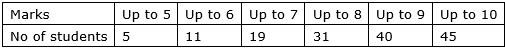The mark wise frequency representation indicates the upper range of the marks and their corresponding frequencies. Let’s form a frequency table showing mark wise frequencies.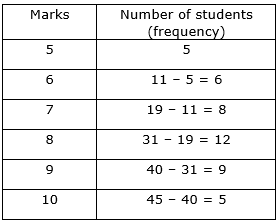We can also represent marks and their corresponding frequencies on a graph, which will show relationship between them.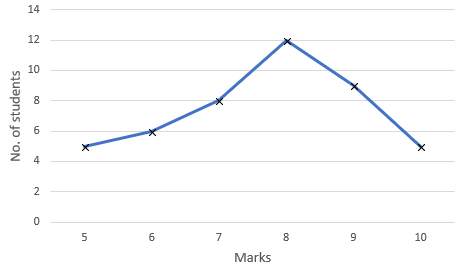Question 2.

Write the mark wise frequencies in the following frequency distribution table.The mark wise frequency representation indicates the upper range of the marks and their corresponding frequencies. Let’s form a frequency table showing mark wise frequencies.We can also represent marks and their corresponding frequencies on a graph, which will show relationship between them.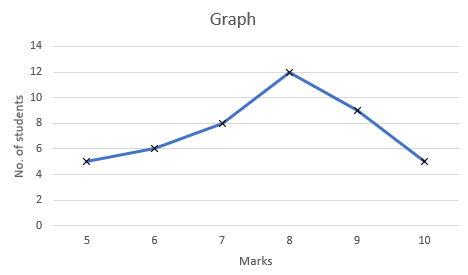Question 3.

The blood groups of 36 students of IX class are recorded as follows.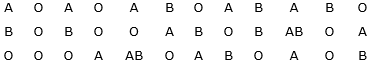Represent the data in the form of a frequency distribution table. Which is the most common and which is the rarest blood group among these students?

Given the blood groups of 36 students can be represented in the form of frequency distribution table.

Just count the occurrence of blood groups in the data and formulate a table.

Count of blood group:

A = 10

B = 9

O = 15

AB = 2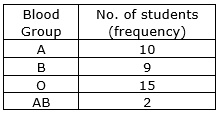Representing the same on a graph to show the relationship between blood group and number of students (frequency),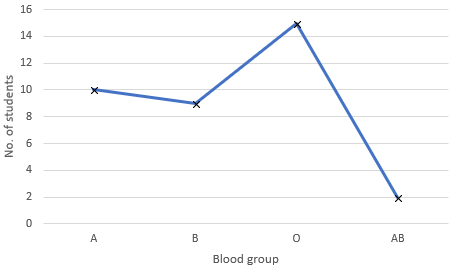From the frequency table as well as from the graph, notice that:

Most common blood group = O [As O has the greatest no. of occurrence, i.e., frequency = 15]

Rarest blood group = AB [As AB has the least no. of occurrence, i.e., frequency = 2]

Question 4.

The blood groups of 36 students of IX class are recorded as follows.

A O A O A B O A B A B O

B O B O O A B O B AB O A

O O O A AB O A B O A O B

Represent the data in the form of a frequency distribution table. Which is the most common and which is the rarest blood group among these students?

Given the blood groups of 36 students can be represented in the form of frequency distribution table.

Just count the occurrence of blood groups in the data and formulate a table.

Count of blood group:

A = 10

B = 9

O = 15

AB = 2Representing the same on a graph to show the relationship between blood group and number of students (frequency),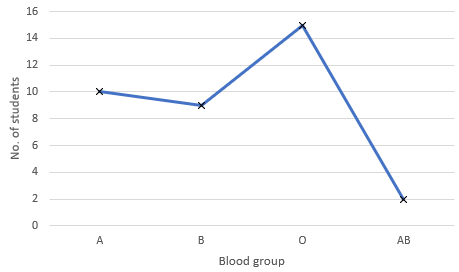From the frequency table as well as from the graph, notice that:

Most common blood group = O [As O has the greatest no. of occurrence, i.e., frequency = 15]

Rarest blood group = AB [As AB has the least no. of occurrence, i.e., frequency = 2]

Question 5.

Three coins were tossed 30 times simultaneously. Each time the number of heads occurring was noted down as follows;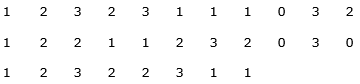Prepare a frequency distribution table for the data given above.

Given the number of heads on tossing three coins can be represented in the form of frequency distribution table.

Just count the occurrence of number of heads in the data and formulate a table.

Count of occurrence of head by 3 coins:

0 (head occurred 0 times) = 3

1 (head occurred 1 times) = 10

2 (head occurred 2 times) = 10

3 (head occurred 3 times) = 7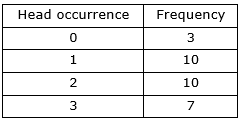It can also be analyzed and represented graphically: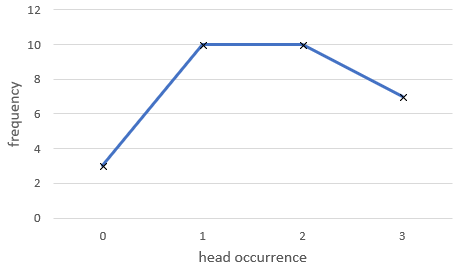Question 6.

Three coins were tossed 30 times simultaneously. Each time the number of heads occurring was noted down as follows;

1 2 3 2 3 1 1 1 0 3 2

1 2 2 1 1 2 3 2 0 3 0

1 2 3 2 2 3 1 1

Prepare a frequency distribution table for the data given above.

Given the number of heads on tossing three coins can be represented in the form of frequency distribution table.

Just count the occurrence of number of heads in the data and formulate a table.

Count of occurrence of head by 3 coins:

0 (head occurred 0 times) = 3

1 (head occurred 1 times) = 10

2 (head occurred 2 times) = 10

3 (head occurred 3 times) = 7It can also be analyzed and represented graphically: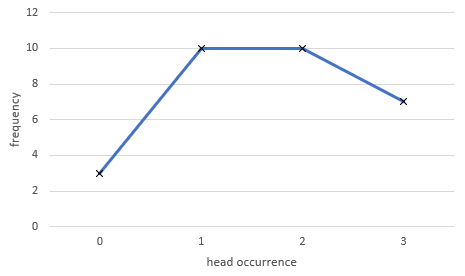Question 7.

A TV channel organized a SMS(Short Message Service) poll on prohibition on smoking, giving options like A – complete prohibition, B – prohibition in public places only, C – not necessary. SMS results in one hour were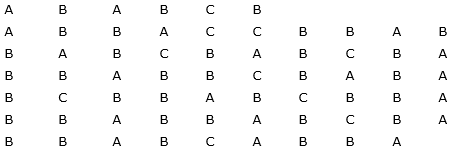Represent the above data as grouped frequency distribution table. How many appropriate answers were received? What was the majority of peoples’ opinion?

Given the poll options (A, B & C) can be represented in the form of frequency distribution table.

Just count the occurrence of each option in the data and formulate a table.

Count of occurrence of options are:

A (complete prohibition) = 19

B (prohibition in public places only) = 36

C (not necessary) = 10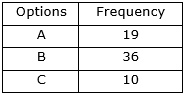It can also be analyzed and represented graphically: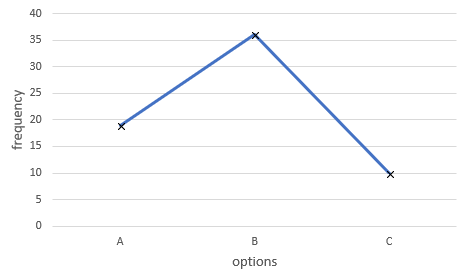That is, 19 + 36 + 10 = 65

To analyze majority votes on poll, mark the highest frequency.

Note that, highest frequency is 36, that is of option B.

And hence, majority of people’s opinion is B, i.e., prohibition in public places only.

Question 8.

A TV channel organized a SMS(Short Message Service) poll on prohibition on smoking, giving options like A – complete prohibition, B – prohibition in public places only, C – not necessary. SMS results in one hour were A B A B C B

A B B A C C B B A B

B A B C B A B C B A

B B A B B C B A B A

B C B B A B C B B A

B B A B B A B C B A

B B A B C A B B A

Represent the above data as grouped frequency distribution table. How many appropriate answers were received? What was the majority of peoples’ opinion?

Given the poll options (A, B & C) can be represented in the form of frequency distribution table.

Just count the occurrence of each option in the data and formulate a table.

Count of occurrence of options are:

A (complete prohibition) = 19

B (prohibition in public places only) = 36

C (not necessary) = 10It can also be analyzed and represented graphically: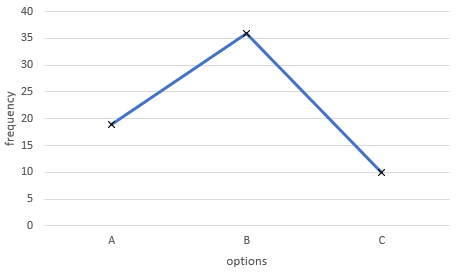That is, 19 + 36 + 10 = 65

To analyze majority votes on poll, mark the highest frequency.

Note that, highest frequency is 36, that is of option B.

And hence, majority of people’s opinion is B, i.e., prohibition in public places only.

Question 9.

Represent the data in the adjacent bar graph as frequency distribution table.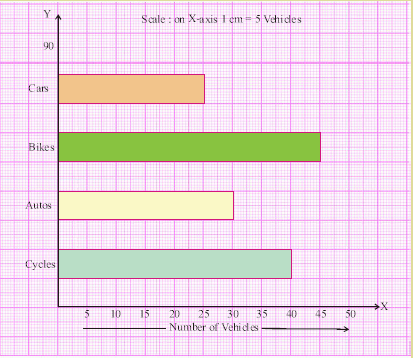Analyzing a bar graph is very easy. Just note down the parameters and its corresponding frequencies by observing the bar graph.

There are 4 parameters for vehicles namely, cycles, autos, bikes and cars.

So now, create a table consisting of these vehicles and their corresponding frequencies.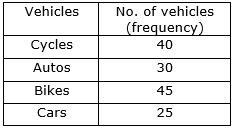Thus, this is the required frequency distribution table.

Question 10.

Represent the data in the adjacent bar graph as frequency distribution table.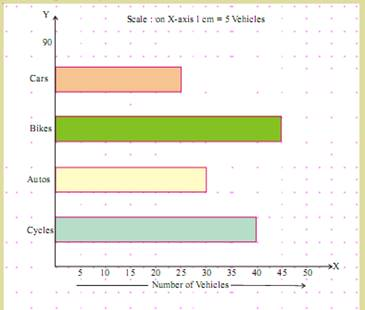Analyzing a bar graph is very easy. Just note down the parameters and its corresponding frequencies by observing the bar graph.

There are 4 parameters for vehicles namely, cycles, autos, bikes and cars.

So now, create a table consisting of these vehicles and their corresponding frequencies.Thus, this is the required frequency distribution table.

Question 11.

Identify the scale used on the axes of the adjacent graph. Write the frequency distribution from it.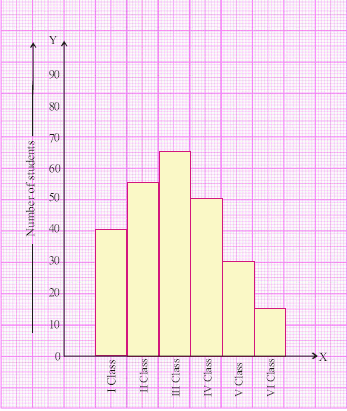Observe that, on x-axis we have classes from I Class to VI Class.

The scale used in x-axis is class on a unit interval.

And on y-axis, we have number of students.

The scale used on y-axis is from 0 to 90 with an interval of 10 units.

Analyzing a histogram is very easy. Just note down the parameters and its corresponding frequencies by observing the bar graph.

There are 6 parameters for classes namely, I Class, II Class, III Class, IV Class, V Class and VI Class.

So now, create a table consisting of these classes and their corresponding frequencies (number of students).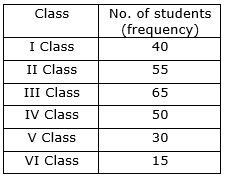Thus, this is the required frequency distribution table.

Question 12.

Identify the scale used on the axes of the adjacent graph. Write the frequency distribution from it.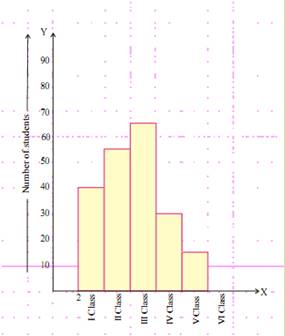Observe that, on x-axis we have classes from I Class to VI Class.

The scale used in x-axis is class on a unit interval.

And on y-axis, we have number of students.

The scale used on y-axis is from 0 to 90 with an interval of 10 units.

Analyzing a histogram is very easy. Just note down the parameters and its corresponding frequencies by observing the bar graph.

There are 6 parameters for classes namely, I Class, II Class, III Class, IV Class, V Class and VI Class.

So now, create a table consisting of these classes and their corresponding frequencies (number of students).Thus, this is the required frequency distribution table.

Question 13.

The marks of 30 students of a class, obtained in a test (out of 75), are given below:

42, 21, 50, 37, 42, 37, 38, 42, 49, 52, 38, 53, 57, 47, 29

59, 61, 33, 17, 17, 39, 44, 42, 39, 14, 7, 27, 19, 54, 51.

Form a frequency table with equal class intervals. (Hint : one of them being 0-10)